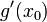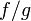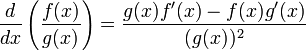# Quotient rule for differentiation

Suppose$f$ and$g$ are functions defined at and around a point$x_0$ and they are both differentiable at$x_0$ (i.e., the derivatives$f'(x_0)$ and$g'(x_0)$ are defined) and$g(x_0) \ne 0$. Then, the quotient$f/g$ is differentiable at$x_0$, and its derivative is given as follows:$\frac{d}{dx}\left(\frac{f(x)}{g(x)}\right)|_{x = x_0} = \frac{g(x_0)f'(x_0) - f(x_0)g'(x_0)}{(g(x_0))^2}$
If we consider the general expressions rather than the evaluation at a particular point$x_0$, we can rewrite the above as:$\frac{d}{dx} \left(\frac{f(x)}{g(x)}\right) = \frac{g(x)f'(x) - f(x)g'(x)}{(g(x))^2}$